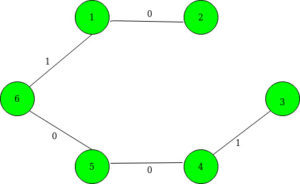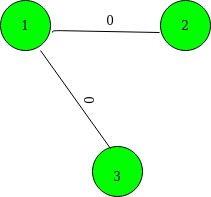# Find weight of MST in a complete graph with edge-weights either 0 or 1

Given an undirected weighted complete graph of N vertices. There are exactly M edges having weight 1 and rest all the possible edges have weight 0. The array arr[][] gives the set of edges having weight 1. The task is to calculate the total weight of the minimum spanning tree of this graph.

Examples:

Input: N = 6, M = 11, arr[][] = {(1 3), (1 4), (1 5), (1 6), (2 3), (2 4), (2 5), (2 6), (3 4), (3 5), (3 6) }
Output: 2
Explanation:
This is the minimum spanning tree of the given graph:Input: N = 3, M = 0, arr[][] { }
Output: 0
Explanation:
This is the minimum spanning tree of the given graph:## Recommended: Please try your approach on {IDE} first, before moving on to the solution.

Approach:
For the given graph of N nodes to be Connected Components, we need exactly N-1 edges of 1-weight edges. Following are the steps:

1. Store the given graph in the map for all the edges of weight 1.
2. Use set to store the vertices which are not included in any of the 0-weight Connected Components.
3. For each vertex currently stored in the set, do a DFS Traversal and increase the count of Components by 1 and remove all the visited vertices during DFS Traversal from the set.
4. During the DFS Traversal, include the 0-weight vertices in a vector and 1-weight vertices in another set. Run a DFS Traversal for all the vertices included in the vector.
5. Then, the total weight of the minimum spanning tree is given the count of components – 1.

Below is the implementation of the above approach:

 `// C++ Program to find weight of ` `// minimum spanning tree in a ` `// complete graph where edges ` `// have weight either 0 or 1 ` `#include ` `using` `namespace` `std; ` ` `  `// To store the edges of the given ` `// graph ` `map<``int``, ``int``> g; ` `set<``int``> s, ns; ` ` `  `// A utility function to perform ` `// DFS Traversal ` `void` `dfs(``int` `x) ` `{ ` `    ``vector<``int``> v; ` `    ``v.clear(); ` `    ``ns.clear(); ` ` `  `    ``// Check those vertices which ` `    ``// are stored in the set ` `    ``for` `(``int` `it : s) { ` `        ``// Vertices are included if ` `        ``// the weight of edge is 0 ` `        ``if` `(!g[x][it]) { ` `            ``v.push_back(it); ` `        ``} ` `        ``else` `{ ` `            ``ns.insert(it); ` `        ``} ` `    ``} ` `    ``s = ns; ` `    ``for` `(``int` `i : v) { ` `        ``dfs(i); ` `    ``} ` `} ` ` `  `// A utility function to find the ` `// weight of Minimum Spanning Tree ` `void` `weightOfMST(``int` `N) ` `{ ` `    ``// To count the connected ` `    ``// components ` `    ``int` `cnt = 0; ` ` `  `    ``// Inserting the initial vertices ` `    ``// in the set ` `    ``for` `(``int` `i = 1; i <= N; ++i) { ` `        ``s.insert(i); ` `    ``} ` ` `  `    ``// Traversing vertices stored in ` `    ``// the set and Run DFS Traversal ` `    ``// for each vertices ` `    ``for` `(; s.size();) { ` ` `  `        ``// Incrementing the zero ` `        ``// weight connected components ` `        ``++cnt; ` ` `  `        ``int` `t = *s.begin(); ` `        ``s.erase(t); ` ` `  `        ``// DFS Traversal for every ` `        ``// vertex remove ` `        ``dfs(t); ` `    ``} ` ` `  `    ``cout << cnt - 1; ` `} ` ` `  `// Driver's Code ` `int` `main() ` `{ ` `    ``int` `N = 6, M = 11; ` `    ``int` `edges[][] = { { 1, 3 }, { 1, 4 }, ` `                      ``{ 1, 5 }, { 1, 6 }, ` `                      ``{ 2, 3 }, { 2, 4 },  ` `                      ``{ 2, 5 }, { 2, 6 },  ` `                      ``{ 3, 4 }, { 3, 5 },  ` `                      ``{ 3, 6 } }; ` ` `  `    ``// Insert edges ` `    ``for` `(``int` `i = 0; i < M; ++i) { ` `        ``int` `u = edges[i]; ` `        ``int` `v = edges[i]; ` `        ``g[u][v] = 1; ` `        ``g[v][u] = 1; ` `    ``} ` ` `  `    ``// Function call find the weight ` `    ``// of Minimum Spanning Tree ` `    ``weightOfMST(N); ` `    ``return` `0; ` `} `

Output:

```2
```

Time Complexity :O(N*log N + M) where N is the number of vertices and M is the number of edges.

Don’t stop now and take your learning to the next level. Learn all the important concepts of Data Structures and Algorithms with the help of the most trusted course: DSA Self Paced. Become industry ready at a student-friendly price.

My Personal Notes arrow_drop_upCheck out this Author's contributed articles.

If you like GeeksforGeeks and would like to contribute, you can also write an article using contribute.geeksforgeeks.org or mail your article to contribute@geeksforgeeks.org. See your article appearing on the GeeksforGeeks main page and help other Geeks.

Please Improve this article if you find anything incorrect by clicking on the "Improve Article" button below.